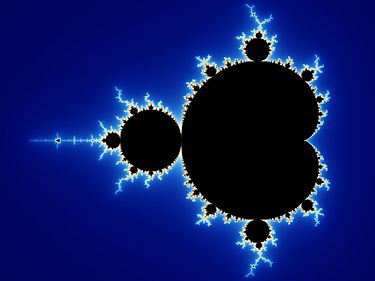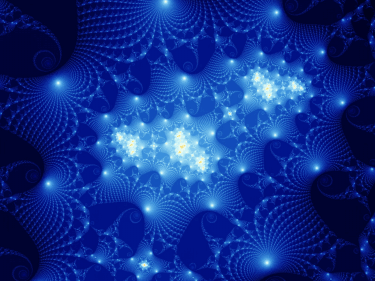Please enable Javascript to view the contents

·  ☕ 4 分钟

## 1. 分形理论

### 1.1 分形的定义### 1.2 分形研究的历史

1975 年开始， 他陆续出版了著作《分形：形状、机遇和维数》和《大自然的分形几何》。曼德波罗的书完全没有得到学术界应有的重视， 直到1982年后，才受到欧美社会的广泛关注，后来被分形学界视为“分形学之圣经”。

## 2. 理解分形

### 2.1 分形的测度维

$$d=log k/log r$$

• 把线段放大两倍后，所得线段可以看成是2个原来的线段叠加而成
• 把正方形放大两倍后，所得正方形可以看成是$$2^2$$个原来的正方形叠加而成
• 把立方体放大两倍后，所得立方体可以看成是$$2^3$$个原来的立方体叠加而成。

### 2.2 Julia集和Mandelbrot集

$${ x_{n+1}={x_n}^2-{y_n}^2+p \\ y_{n+1}=2x_ny_n+q }$$

Julia 集的绘制方法：

1. 设定初值 p,q, 最大的迭代次数 N, 图形的大小 a,b, 及使用的颜色数 K.这里需要注意的是c的模总是小于2。可以证明当c的模大于2时，进行迭代必将发散到无穷。
2. 设定区域的界值 $$M\ge max(2,\sqrt{p^2+q^2})$$
3. 将区域$$R=[-M,M]\times[-M,M]$$分成$$a\times b$$的网格，分别以每个网格点为初值($$x_0,y_0$$)。利用上面替换之后的公式做迭代。如果对$$n \le N$$所有的都有$${x_n}^2+{y_n}^2\le M^2$$，则将象素$$(i, j)$$置为这一种颜色。如果从某一步 n 开始$${x_n}^2+{y_n}^2\ge M^2$$，则将象素 $$(i, j)$$置为不同颜色。

Mandelbrot集的绘制方法：

## 3. 参考